Topics: AI | Data Science | Data Visualization | Deep Learning | Machine Learning | NLP | Python | R | Statistics

KDnuggets Home » News » 2017 » Aug » Tutorials, Overviews » Support Vector Machine (SVM) Tutorial: Learning SVMs From Examples

# Support Vector Machine (SVM) Tutorial: Learning SVMs From Examples

http likes 143

In this post, we will try to gain a high-level understanding of how SVMs work. I’ll focus on developing intuition rather than rigor. What that essentially means is we will skip as much of the math as possible and develop a strong intuition of the working principle.

### Non-linearly Separable Data

We have seen how Support Vector Machines systematically handle perfectly/almost linearly separable data. How does it handle the cases where the data is absolutely not linearly separable? Afterall, a lot of real-world data falls in this category. Surely, finding a hyperplane can’t work anymore. This seems unfortunate given that SVMs excel at this task.

Here’s an example of non-linearly separable data (this is a variant of the famous XOR dataset), shown with the linear classifier SVMs find: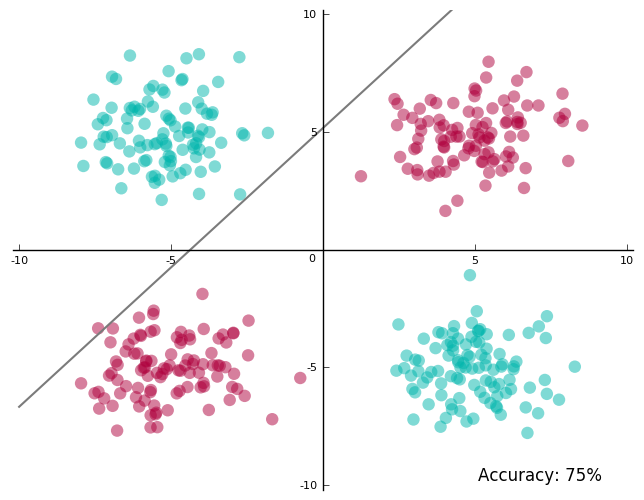You’d agree this doesn’t look great. We have only 75% accuracy on the training data — the best possible with a line. And more so, the line passes very close to some of the data. The best accuracy is not great, and to get even there, the line nearly straddles a few points.

We need to do better.

This is where one of my favorite bits about SVMs come in. Here’s what we have so far: we have a technique that is really good at finding hyperplanes. But then we also have data that is not linearly separable. So what do we do? Project the data into a space where it is linearly separable and find a hyperplane in this space!

I’ll illustrate this idea one step at a time.

We start with the dataset in the above figure, and project it into a three-dimensional space where the new coordinates are: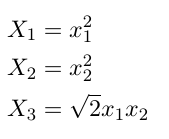This is what the projected data looks like. Do you see a place where we just might be able to slip in a plane?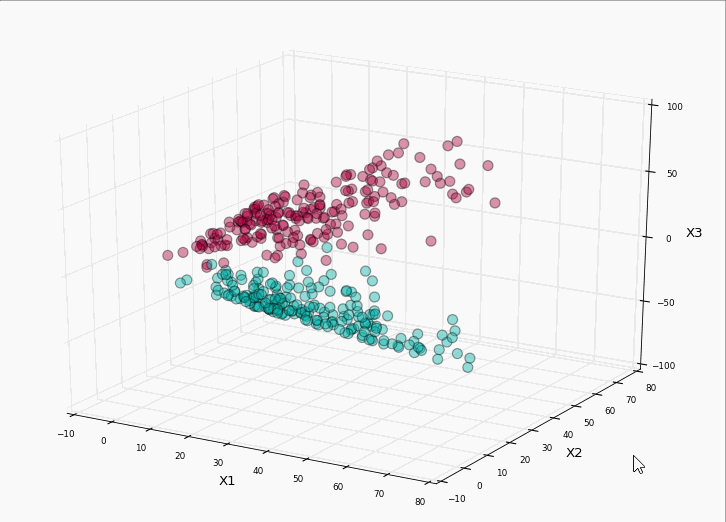Let’s run our SVM on it: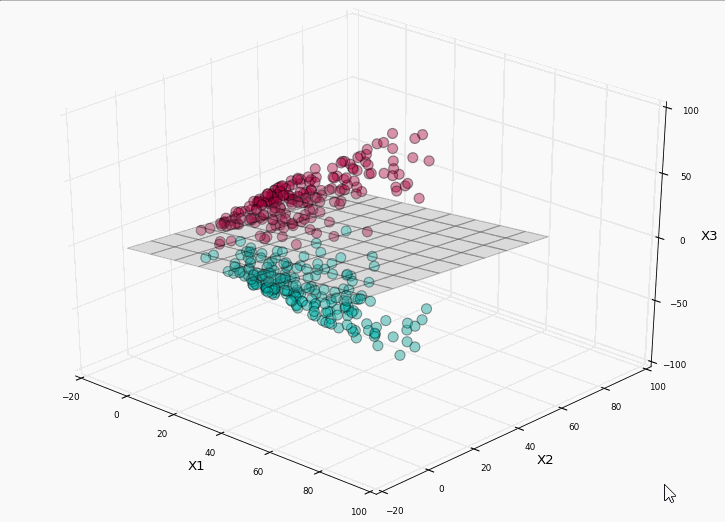Bingo! We have perfect label separation! Lets project the plane back to the original two-dimensional space and see what the separation boundary looks like: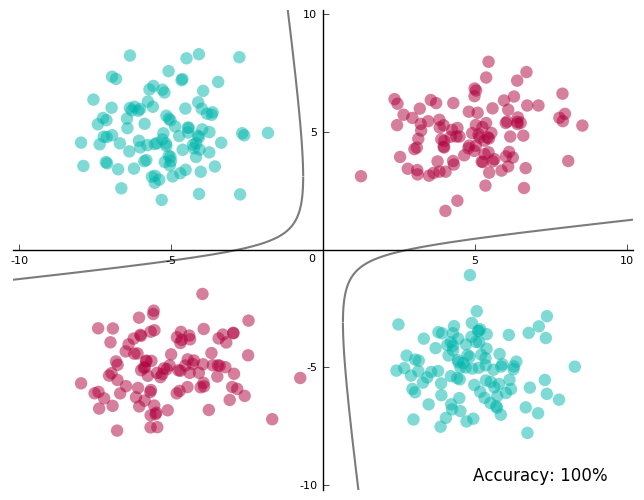100% accuracy on the training data and a separating boundary that doesn’t run too close to the data! Yay!

The shape of the separating boundary in the original space depends on the projection. In the projected space, this is always a hyperplane.

Remember the primary goal of projecting the data was to put the hyperplane-finding superpowers of SVMs to use.

When you map it back to the original space, the separating boundary is not a line anymore. This is also true for the margin and support vectors. As far as our visual intuition goes, they make sense in the projected space.

Take a look at what they look like in the projected space, and then in the original space. The 3D margin is the region (not shaded to avoid visual clutter) between the planes above and below the separating hyperplane.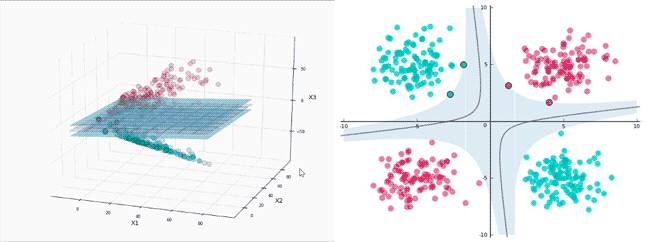There are 4 support vectors in the projected space, which seems reasonable. They sit on the two planes that identify the margin. In the original space, they are still on the margin, but there doesn’t seem to be enough of them.

Let’s step back and analyze what happened:

1. How did I know what space to project the data onto?

It seems I was being utterly specific — there is a square root of 2 in there somewhere!

In this case, I wanted to show how projections to higher dimensions work, so I picked a very specific projection. In general, this is hard to know. However, what we do know is data is more likely to be linearly separable when projected onto higher dimensions, thanks to Cover’s theorem.

In practice, we try out a few high-dimensional projections to see what works. In fact, we can project data onto infinite dimensions and that often works pretty well. This deserves going into some detail and that’s what the next section is about.

2. So I project the data first and then run the SVM?

No. To make the above example easy to grasp I made it sound like we need to project the data first. The fact is you ask the SVM to do the projection for you. This has some benefits. For one, SVMs use something called kernels to do these projections, and these are pretty fast (for reasons we shall soon see).

Also, remember I mentioned projecting to infinite dimensions in the previous point? If you project the data yourself, how do you represent or store infinite dimensions? It turns out SVMs are very clever about this, courtesy of kernels again.

It’s about time we looked at kernels.Get KDnuggets, a leading newsletter on AI, Data Science, and Machine Learning